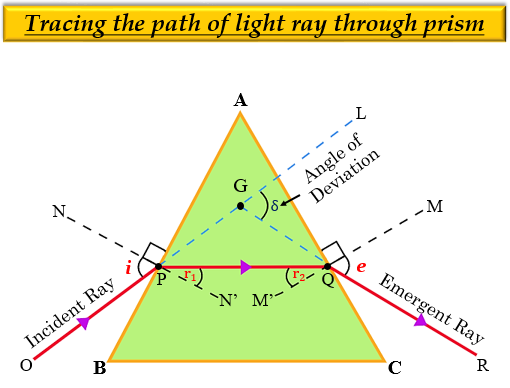# In an experiment to trace the path of a ray of light through a glass prism for different values of angle of incidence a student would find that the emergent ray:(a) is parallel to the incident ray.(b) perpendicular to the incident ray.(c) is parallel to the refracted ray.(d) bends at an angle to the direction of the incident ray.

(d) bends at an angle to the direction of the incident ray.

Explanation

In an experiment to trace the path of a ray of light through a glass prism for different values of angle of incidence a student would find that the emergent ray bends at an angle to the direction of the incident ray. This happens because the light ray gets refracted two times at different angles. So, the emergent rays bend at an angle towards the direction of the incident ray.

It can be shown with the help of the following diagram:Updated on: 10-Oct-2022

694 Views